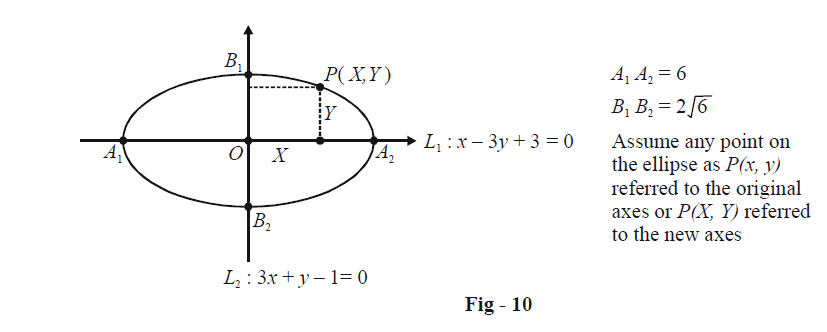# Basic Examples On Ellipses Set-1

Go back to  'Ellipse'

Example - 2

Find the equation of the ellipse with centre at the origin, foci at$$( \pm 1,\,\,0)$$ and $$e = \frac{1}{2}.$$

Solution: Let the major axis be of length 2a. The distance between the two foci, 2ae, is equal to 2 for this example.

\begin{align}&\qquad\qquad2ae = 2\\&\Rightarrow\quad a = \frac{1}{e} = 2\end{align}

Thus,

$b = a\sqrt {(1 - {e^2})} = \sqrt 3$

The equation of the ellipse is therefore

$\frac{{{x^2}}}{4} + \frac{{{y^2}}}{3} = 1$

Example - 3

Find the equation of the ellipse whose major and minor axes lie along the lines $$x - 3y + 3 = 0{\rm{\; }}and{\rm{\; }}3x + y - 1 = 0$$ and whose lengths are 6 and $$2\sqrt 6$$ respectively.

Solution: The equation to the ellipse will obviously not be in the standard form since the axes are not along the coordinate axes. However, we can use the coordinate axes formed by these two lines as our reference frameConsider an arbitrary point P on the ellipse whose coordinates are (x, y) with respect to the original axes (not shown) and (X, Y) with respect to the new axes, the L1 – L2 system. In this new system, the equation of the ellipse is simply

$\frac{{{X^2}}}{{{a^2}}} + \frac{{{Y^2}}}{{{b^2}}} = 1$

where a = 3 and $$b = \sqrt 6 .$$ Thus, the equation is

$\frac{{{X^2}}}{9} + \frac{{{Y^2}}}{6} = 1\qquad\qquad\qquad...\left( 1 \right)$

Now, we wish to write the equation of the ellipse in the x-y coordinate system. For this purpose, we make the following observations from the figure:

What is X? It is simply the perpendicular distance of P from L2:

$X = \frac{{\left| {3x + y - 1} \right|}}{{\sqrt {10} }}$

Similarly, Y is simply the perpendicular distance of P from L1 :

$Y = \frac{{\left| {x - 3y + 3} \right|}}{{\sqrt {10} }}$

Thus, using (1), the equation of the ellipse in x-y form is

\begin{align}&\frac{{{{(3x + y - 1)}^2}}}{{90}} + \frac{{{{(x - 3y + 3)}^2}}}{{60}} = 1\\&\Rightarrow 21{x^2} - 6xy + 29{y^2} + 6x - 58y - 151 = 0\\\end{align}

As an exercise find the centre and the foci of this ellipse.

(Hint: The centre is simply the intersection of Land L2. The foci are at $$( \pm ae,\,\,0)$$ in the X -Y system. To find the foci in the x-y system, find the two points along L1 which are at a distance of ae from O on either side of it)

Although we will not have much use for it, it is still worth mentioning that given an arbitrary fixed point P(h, k) and an arbitrary fixed straight line $$lx + my + n = 0$$ as the focus and directrix of an ellipse with eccentricity e, its equation can be written by using the definition of an ellipse. Let (x, y) be any point on the ellipse:

\begin{align}&\frac{{{\rm{Distance \;of\; (}}x{\rm{, }}y{\rm{)\; from\; }}P}}{{{\rm{Distance \;of\; (}}x{\rm{, }}y{\rm{)\; from\; the\; line }}}} = e\\&\Rightarrow {(x - h)^2} + {(y - k)^2} = {e^2}\frac{{{{(lx + my + n)}^2}}}{{{l^2} + {m^2}}}\end{align}

This gives us the general equation of an ellipse with a given eccentricity, focus and directrix. However, we will almost always be using the (simple !) standard form of the equation.